Bandwagon (full documentation here) is a Python library to predict and plot migration patterns from DNA digestions. It supports hundreds of different enzymes (thanks to BioPython), single- and multiple-enzymes digestions, and custom ladders.

It uses Matplotlib to produce plots like this one: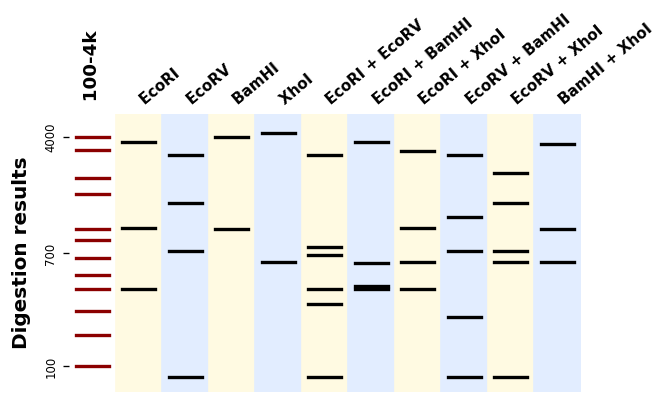# Bandwagon¶

Bandwagon is an open-source software originally written at the Edinburgh Genome Foundry by Zulko and released on Github under the MIT licence (Copyright Edinburg Genome Foundry).

Everyone is welcome to contribute !

## Installation¶

If you have pip installed, just type:

```(sudo) pip install bandwagon
```

Bandwagon can be installed by unzipping the source code in one directory and using this command:

```(sudo) python setup.py install
```

To create interactive bokeh plots you will need to additionally install Bokeh with

```(sudo) pip install bokeh
```

## Examples of use¶

### Computing digestion bands sizes¶

This first example shows how to compute digestion bands in the case of a linear fragment, a circular fragment, and a multi-enzymes digestion:

```from bandwagon import compute_digestion_bands

# Read the sequence (a string of the form 'ATGTGTGGTA...' etc.)
with open("example_sequence.txt", "r") as f:

# Compute digestion bands for a linear construct
print(compute_digestion_bands(sequence, ["EcoRI"], linear=True))
# Result >>> [400, 1017, 3583]

# Compute digestion bands for a circular construct
print(compute_digestion_bands(sequence, ["EcoRI"], linear=False))
# Result >>> [1017, 3983]

# Compute digestion bands for an enzymatic mix
print(compute_digestion_bands(sequence, ["EcoRI", "BamHI"]))
# Result >>> [400, 417, 600, 3583]
```

### Plotting bands¶

```from bandwagon import BandsPattern, BandsPatternsSet, LADDER_100_to_4k

patterns = [
BandsPattern([100, 1200, 1400, 3000], ladder, label="C3"),
]
ax = patterns_set.plot()
ax.figure.savefig("simple_band_patterns.png", bbox_inches="tight", dpi=200)
```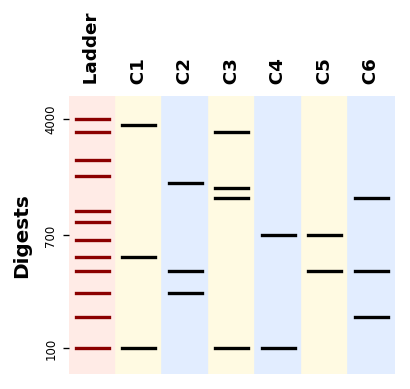### Plotting a gel simulation¶

Let us plot digestion patterns produced by different restriction enzymes on a same DNA sequence:

```from bandwagon import (BandsPattern, BandsPatternsSet, LADDER_100_to_4k,
compute_digestion_bands)

with open("example_sequence.txt", "r") as f:

patterns = [
BandsPattern(compute_digestion_bands(sequence, [enzyme], linear=True),
for enzyme in ["BamHI", "EcoRI", "EcoRV", "PstI", "SpeI", "XbaI"]
]

ax = patterns_set.plot()
ax.figure.savefig("digestion_results.png", bbox_inches="tight", dpi=200)
```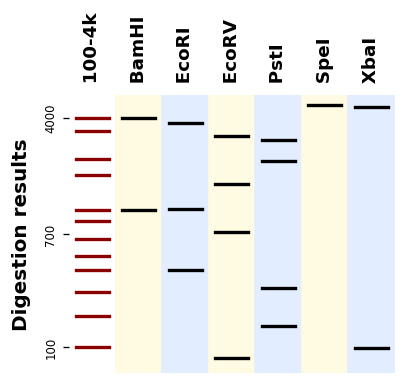If you have many sequences and digestions you can also use the utility `plot_records_digestions`

```from bandwagon import plot_all_digestion_patterns, LADDER_100_to_4k

plot_records_digestions(
records=records,
digestions=[('BamHI', 'NcoI'), ('BsaI', 'XbaI'), ('StyI',)],
target="records_digestions.pdf")

axes = plot_all_digestion_patterns(
records=records,
digestions=[('BamHI', 'NcoI'), ('BsaI', 'XbaI'), ('StyI',)],
)
axes.figure.savefig("plot_all_digestion_patterns.png")
```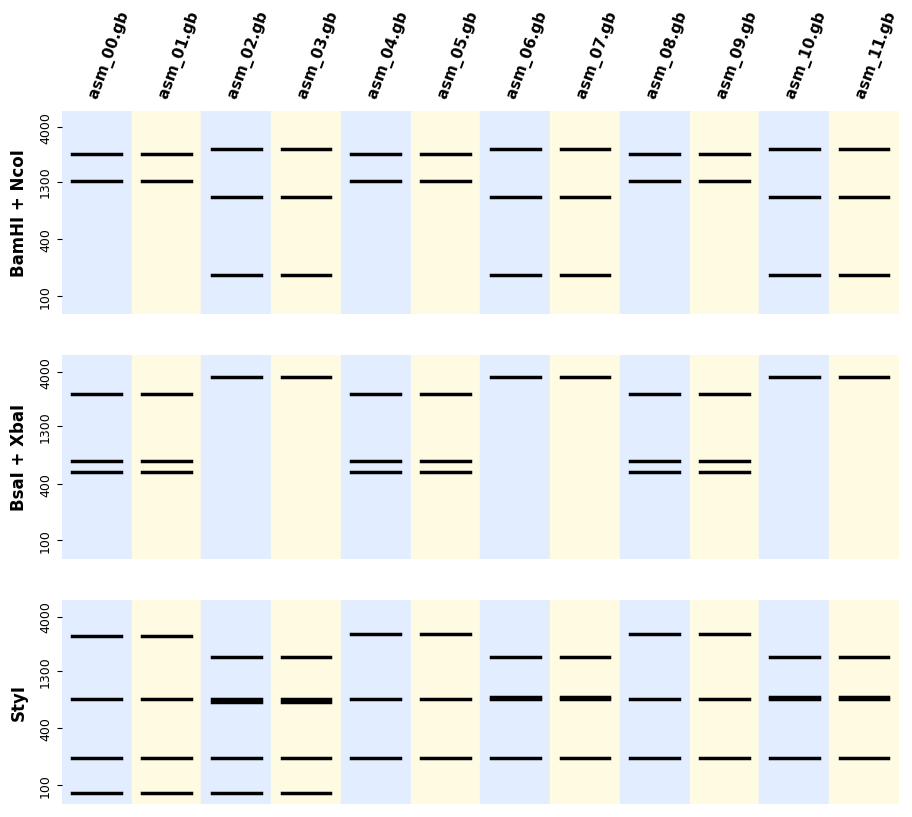### Plotting patterns alongside annotated records¶

You can also get a full report with indications of where in your sequences the bands are formed (which is useful for troubleshooting) as follows:

```from bandwagon import plot_records_digestions, LADDER_100_to_4k

plot_records_digestions(
records=records,
digestions=[('BamHI', 'NcoI'), ('BsaI', 'XbaI'), ('StyI',)],
target="records_digestions.pdf")
```

You get a PDF report with one page per construct and digestion, looking like this: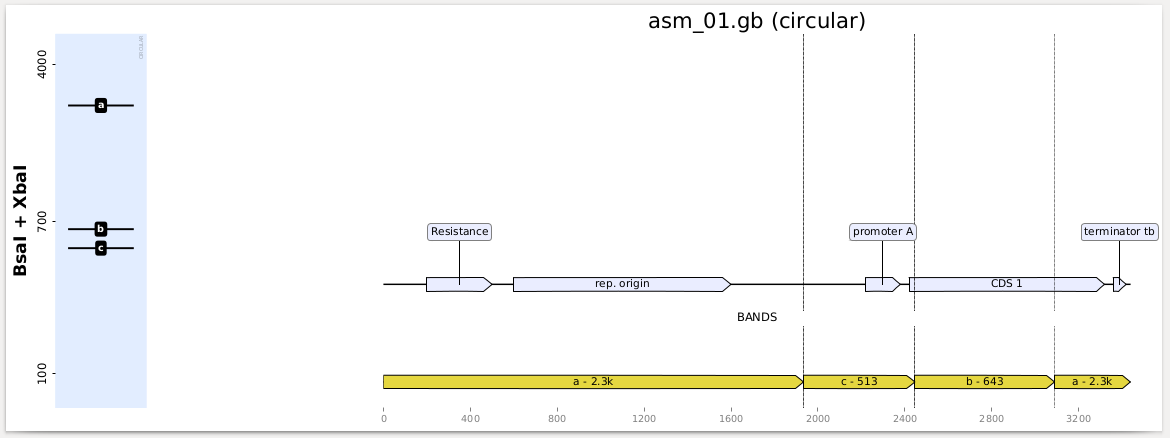You can define a custom ladder by providing a dictionnary of the form

```{ actual_size_of_the_fragment: observed_migration_distance }
```

For instance here is how the 100b-4kb ladder (provided with BandWagon) is defined:

```from bandwagon import custom_ladder

100: 205,
200: 186,
300: 171,
400: 158,
500: 149,
650: 139,
850: 128,
1000: 121,
1650: 100,
2000: 90,
3000: 73,
4000: 65
})
```

The unit of the “migration distance” from the starting point is not very important, it could be millimeters on a gel, pixels in an image, etc.

If you are lucky enough to have an AATI automated fragment analyzer like us at the Foundry, it will output a `.csv` calibration file after each run, from which you can generate a ladder with:

```from bandwagon import ladder_from_aati_fa_calibration_table